# Control function

control

A function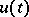occurring in a differential equation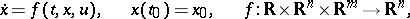(1)

and the values of which at each moment of time may be chosen arbitrarily.

Usually the domain of variation of, for each, is subject to a restriction(2)

whereis a given closed set in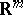. A control is called admissible if for eachit satisfies the constraint (2). Different admissible controlsdefine corresponding trajectories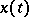, starting from the initial point.

If one is given a functional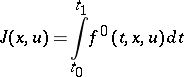(3)

and a boundary condition at the right-hand end of the trajectory at: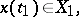(4)

whereis a certain set in(in a special case, a single point), then one may raise the question of determining an optimal control, giving an optimal value for the functional in the problem (1)–(4). Questions connected with the definition of optimal control functions have been considered in the theory of optimal control and variational calculus (cf. , ).

In distinction to the variables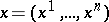, called phase variables (or phase coordinates), the controloccurs in equation (1) without derivatives. Therefore (1) has a meaning not only for continuous, but also for piecewise-continuous (and even measurable) controls. Moreover, for each admissible piecewise-continuous controlequation (1) defines a continuous piecewise-smooth trajectory.

In the majority of problems an optimal control exists in the class of piecewise-continuous functions. However, one meets with problems in which the optimal control is not piecewise continuous, but belongs to the class of Lebesgue-measurable functions. In these cases the optimal control has an infinite number of points of discontinuity, approaching a certain point, for example, an entry point for a singular surface of order,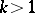(cf. Optimal singular regime).

Necessary conditions, formulated in the theory of optimal control in the form of Pontryagin's maximum principle, have been proved in the general case in which the optimal control function being studied is assumed measurable (in particular, it may be piecewise continuous or continuous).

According to the maximum principle, forto be an optimal control (in the case of minimization of the functional (3)) it is necessary that for eachthe control gives a maximum of the Hamiltonian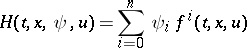on, whereis a conjugate vector function, defined by the conjugate systemAn analogous maximum principle in the calculus of variations allows one to define "free" functions under constraints given by the Euler equations and the Weierstrass necessary condition.

For the existence of an optimal Lebesgue-measurable control giving a minimum of the functional (3) it is sufficient that for each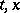the set of values of the vector on the right-hand side,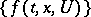, obtained as the controlranges over all admissible values of the domain, is convex in, and that the greatest lower bound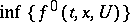of the set of values assumed by the integrand is convex from below as a function in. If this condition is not fulfilled, there may be cases where the minimizing sequence of controls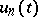no longer converges, even in the class of measurable functions. In these cases one says that one has a sliding (or relaxed) optimal regime (control). A sliding optimal control may be given in a strict sense as a singular optimal control in a related problem closely connected with the initial one (cf. Optimal sliding regime).

Using the necessary as well as the sufficient conditions for optimality established in the theory of optimal control and the calculus of variations one may define optimal control functions and the corresponding optimal phase trajectories in the problems being considered. There are different numerical methods for constructing optimal controls (cf. Variational calculus, numerical methods of).

In more general cases the control function may depend on several arguments. In that case one speaks of a control function with distributed parameters.

How to Cite This Entry:
Control function. Encyclopedia of Mathematics. URL: http://encyclopediaofmath.org/index.php?title=Control_function&oldid=16659